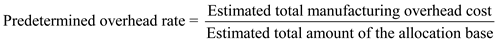# Quiz 3: Job-Order Costing: Cost Flows and External Reporting

Business

Predetermined Overhead rate: Predetermined overhead rate is useful in applying manufacturing overhead to work in process inventory. Usually, this predetermined overhead rate is calculated at the beginning of a period. This rate is used to charge overhead costs to job in production. Predetermined overhead rate is calculated by using the following formula:The predetermined overhead can be computed in two steps. First, calculate the estimated overhead. Second, use the estimated overhead in the calculation of the predetermined overhead. The estimated total manufacturing overhead cost is computed as follows:The predetermined overhead rate is computed as follows:Therefore, predetermined overhead rate is \$14.65 per direct labour hour.

By definition, manufacturing overhead consists of costs that cannot be practically traced to jobs. Therefore, if these costs are to be assigned to jobs, they must be allocated rather than traced.

The formula for manufacturing overhead applied is: